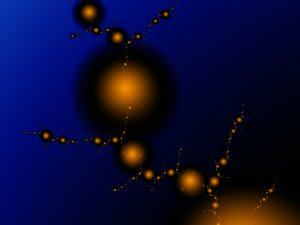A# fractalA fractal is a geometric shape that can be subdivided at any scale into parts that are, at least approximately, reduced-size copies of the whole. The name "fractal," from the Latin fractus meaning a broken surface, was coined by Benoit Mandelbrot in 1975. The key property of fractals is self-similarity, which means that zooming in or zooming out of a fractal produces no overall change in appearance.

One of several technical definitions of a fractal is "a set of points whose topological dimension is less than its Hausdorff dimension". The topological dimension is an object's ordinary dimensionality – one in the case of a curve, two in the case of a surface, and so – and is always a whole number. The Hausdorff dimension, on the hand, measures how much space an object fills, and can take non-integer values if the object is very complex and twisty.

Some fractals show a strong regularity and rigid self-similarity and are produced by the repeated application of a set of rules that may be quite simple. Among the best known of these "iterated function" systems are the Koch snowflake, the Peano curves, the Sierpinski carpet, and the Sierpinski gasket. Other fractals, defined by a recurrence relation at each point in space, are among the most complex, beautiful, and beguiling mathematical structures known. They include the well-known Mandelbrot set and Lyapunov fractals. Finally, there are random fractals generated by stochastic rather than deterministic processes, for example fractal landscapes. Random fractals have the greatest practical use, and can be used to describe many highly irregular real-world objects, including clouds, mountains, coastlines and trees.

## Fractal dimension

A fractal dimension is a non-integer measure of the irregularity or complexity of a system; it is an extension of the notion of dimension found in Euclidean geometry. Knowing the fractal dimension helps one determine the degree of irregularity and pinpoint the number of variables that are key to determining the dynamics of the system.